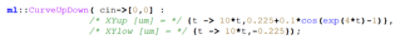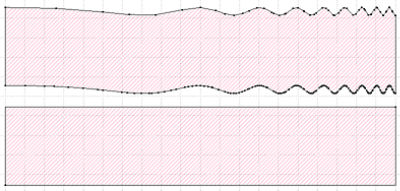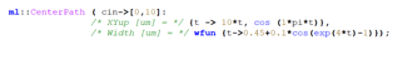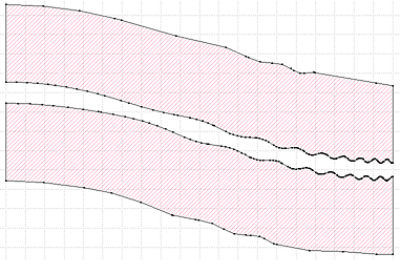# Feature Highlight: Arbitrary Curves and Width Profiles

## Introduction

When creating a PIC layout people mostly use simple components like pre-configured PDK components,  simple straights or polar bends. However the Synopsys PIC Design Suit layout tool OptoDesigner (OD) is capable of creating any desired shape, while discretizing it to mask files accurately, which gives a broad range of design freedom.

Waveguide shapes – even simple ones like straights – in OptoDesigner consist of two curves; an ‘upper’ curve and a ‘lower’ curve, when looking at it running left-to-right. Each layer associated to the active mask cross-section has its own ‘upper’ and ‘lower’ curve, which are related to the original curves – usually either directly or through widening. These are all analytic curves. When exporting to a mask file, these curves are turned into polygons. The rules in the OD PDK (Process Design Kit) determine how accurately this happens; the curve is analyzed, and an algorithm makes sure that all vertices of the mask polygons lie within a given distances from the analytical curve (typically 1 nm). Note that this discretization into polygons is still done with full precision; a snap to the mask file grid will introduce extra errors. Since this algorithm happens for any waveguide shape, it also enables accurate discretization of fully arbitrary shapes.

The OptoDesigner Element category ‘Curves’ contains a number of different ways of creating arbitrary curves. This Feature Highlight will dive into two of those: CurveUpDown and CenterPath.

## CurveUpDown

CurveUpDown has two arguments – XYup and XYlow. Each of these is a function of a parameter t, which runs from 0 to 1, and describes a curve through space. The input port, cin, is located in the middle between the XYup and XYlow curves at t=0; the output port, cout, lies in the middle between XYup and XYlow at t=1. Their respective angles are the averages of the angles of the two curves.

unction arguments in the script are written at { t -> sin(t) } for a scalar function, or { t -> sin(t), cos(t) } for a vector function. An example of a CurveUpDown that implements a one-sided grating whose period exponentially decreases is:When we use this for the silicon photonics waveguide type in the SOI DemoFab, the mask that gets generated looks like:Note that since this waveguide type is formed by trenches instead of the waveguide core itself, the tool automatically created these layers from the curve description. Also note that the density of vertices of the polygons is higher where the curve is more difficult, in order to keep the vertices within a given distance from the analytical curve. Also note that PDK specifies that the outer edge of the trench is allowed 10 times more distance between the curve and the polygon, and thus is discretized much more coarsely.

## CenterPath

The CenterPath is similar to the CurveUpDown. It is a suitable for curves that have a well-defined center path, with some width around it. Its first argument is the curve for the center path; the second is a width definition, which can be a built-in function like fixed or linear, or a user-defined function. The example below creates a cosine-shaped path with the same grating shape as above – except this time symmetrical:The generated shapes look like:Again, the polygons are much denser where the curve is difficult, and the outer edges are discretized much more coarsely than the inner edges.

## Pro Tip

Using these script functions to create shapes is very flexible but can severely slow down mask export. This is caused by the discretization algorithm needing to execute a script function each time it needs to know the location of the curves, which is slow. Using ‘functors’, this can be sped up immensely. Functors look like script functions, but they are converted to c++ objects that evaluate orders of magnitude more quickly than normal script functions. Functors are written like { functor = sin(#) } for a scalar function, or { functor = sin(#), cos(#) } for a vector function. The ‘#’ character takes the place of the running variable ‘t’. The two curves then look like this: